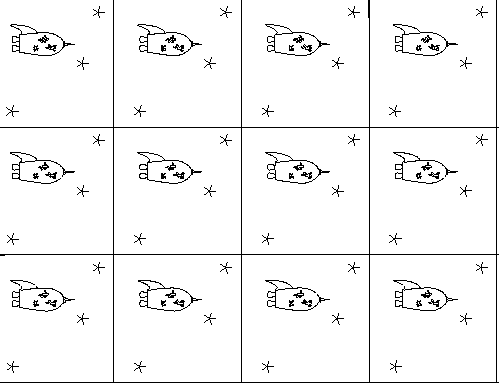### Project 4

You may work in a group of 4 people maximum, or work alone.

• Problem 1 p. 73 Exercise 2.1.13 and some additional directions: Part A: By a patch, the book means local coordinates. In order to examine the role of u and v, hold one constant and think about what kind of curve the other gives. This is an extrinsic definition. While it is not listed, R is the distance away from the origin.

Part B: In addition, compute Xu x Xv and show that it is never the 0 vector. What does this tell you about regularity and the existence of that tangent plane?

Part C: Compute F=Xu · Xv. Interpret your result, ie what does this tell you about the relationship between Xu and Xv.

For parts B and C, you may wish to use the following commands and procedures in Maple:
Xu := [diff(X,u),diff(X,u),diff(X,u)];
Xv := [diff(X,v),diff(X,v),diff(X,v)];

xp := proc(X,Y)
local a,b,c;
a := X*Y-X*Y;
b := X*Y-X*Y;
c := X*Y-X*Y;
[a,b,c];
end:

dp := proc(X,Y)
X*Y+X*Y+X*Y;
end:

Part D: Open up the Maple demo that is accessible from the main web page, and input the torus instead of the sphere. Choose specific values for r and R. Explore and find two different curves on the your specific (r,R) torus - one that is a geodesic and one that is not. For each curve:
Part D(1): Provide your new values for each of the following (these are the commands I used for the sphere):
g := (x,y) -> [cos(x)*sin(y), sin(x)*sin(y), cos(y)]:
a1:=0: a2:=Pi: b1:=0: b2:=Pi:
c1 := 1: c2 := 3:
Point := 2:
f1:= (t) -> t:
f2:= (t) -> 1:
Part D(2): Sketch by-hand or print out a picture of the curvatures and the torus.
Part D(3): Discuss your curves from an intrinsic point of view - ie why is the geodesic a geodesic, and why is the other curve not "straight." You should refer to an intrinsic argument - ie symmetries, the ribbon test, and/or a covering space argument.

Part E: You have already calculated F. Calculate E and G. Set up a double integral that uses the metric coefficients that could be used to find the surface area of the torus. Explain your limits of integration.

• Problem 2 Read Example 5.4.9 beginning on p. 229. Part A: Calculate E, F and G and show work.

Part B: Next examine the metric form (ds/dt)2 and use this to discuss whether the Pythagorean theorem holds on this surface.

Part C: The flat torus can be obtained by taking a square and identifying the edges straight across (top to bottom and separately left to right). So a covering space would be infinitely squares next to each other which are exact copies of each other:How many geodesics join two points? Explain and draw pictures in the covering space.

Part D: Can a geodesic on the flat torus ever intersect itself? Explain and draw pictures in the covering space.